Search test library by skills or roles
⌘ K

Here are some sample Tableau questions from our premium questions library (10273 non-googleable questions).

### Skills

#### Accounting

🧐 Question

Medium

Calculated Properties
Data Analysis
Solve
`Here’s sample data imported into Tableau:`
```Assume only three rows of data is present in the source. Once the data is imported, we added to new calculated fields:

Measure 1 with the formula: SUM([Profit])/SUM([Sales])
Measure 2 with the formula: SUM([Profit]/[Sales])

If we dragged Profit, Sales, Measure 1 and Measure 2 in the viewer as shown here:```
`Pick the correct statements regarding columns Measure 1, Measure 2 and the row Grand total:`
```A: Values of Measure 1 would be 0.08, 0.075 and 0.1
B: Values of Measure 2 would be 0.08, 0.075 and 0.1
C: Values of Measure 1 would be 0.08, 0.769 and 0.08
D: Values of Measure 2 would be 0.08, 0.769 and 0.08
E: Values of Measure 1 would be 0.08, 0.155 and 0.255
F: Values of Measure 2 would be 0.08, 0.155 and 0.255
G: Grand total of Measure 1 would be 0.08
H: Grand total of Measure 2 would be 0.08
I: Grand total of Measure 1 would be 0.255
J: Grand total of Measure 2 would be 0.255```

Medium

Change display icon
Solve
`What steps would you take to change the following view so that Office Supplies is represented by a different symbol?`
```A: Click on shapes in the marks area. Then select Office Supplies and the icon of choice and click okay.
B: Right-click on product category, select default properties, and select shape. Then select Office Supplies and the icon of choice and click okay.
C: Right click on the square and select "change shape" from the popup menu. Select the icon of choice and click okay.
D: A, B or C
E: None of these would work```

Easy

Pie Chart
Data Analysis
Charts
Solve
`We have the following dataset in Tableau:`
`Which of the following Marks settings will result in following Pie chart?`
```A: Drag Name to Color
B: Drag Share to Color
C: Drag Name to Label
D: Drag Share to Label
E: Drag Name to Angle
F: Drag Share to Angle
G: Drag Name to Detail```
🧐 Question🔧 Skill

Medium

Calculated Properties
Data Analysis
3 mins
Tableau
Solve

Medium

Change display icon
2 mins
Tableau
Solve

Easy

Pie Chart
Data Analysis
Charts
3 mins
Tableau
Solve
🧐 Question🔧 Skill💪 Difficulty⌛ Time
Calculated Properties
Data Analysis
Tableau
Medium3 mins
Solve
Change display icon
Tableau
Medium2 mins
Solve
Pie Chart
Data Analysis
Charts
Tableau
Easy3 mins
Solve

## Adaface questions are trusted by enterprises globally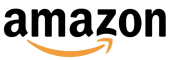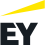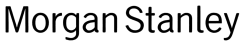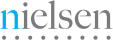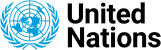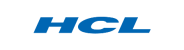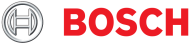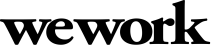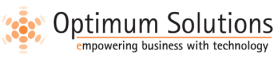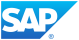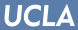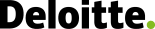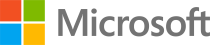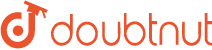We evaluated several of their competitors and found Adaface to be the most compelling. Great default library of questions that are designed to test for fit rather than memorization of algorithms.

Swayam Narain, CTO, AffableJoin 1200+ companies in 75+ countries.
Essayez l'outil d'évaluation des compétences le plus candidat aujourd'hui.